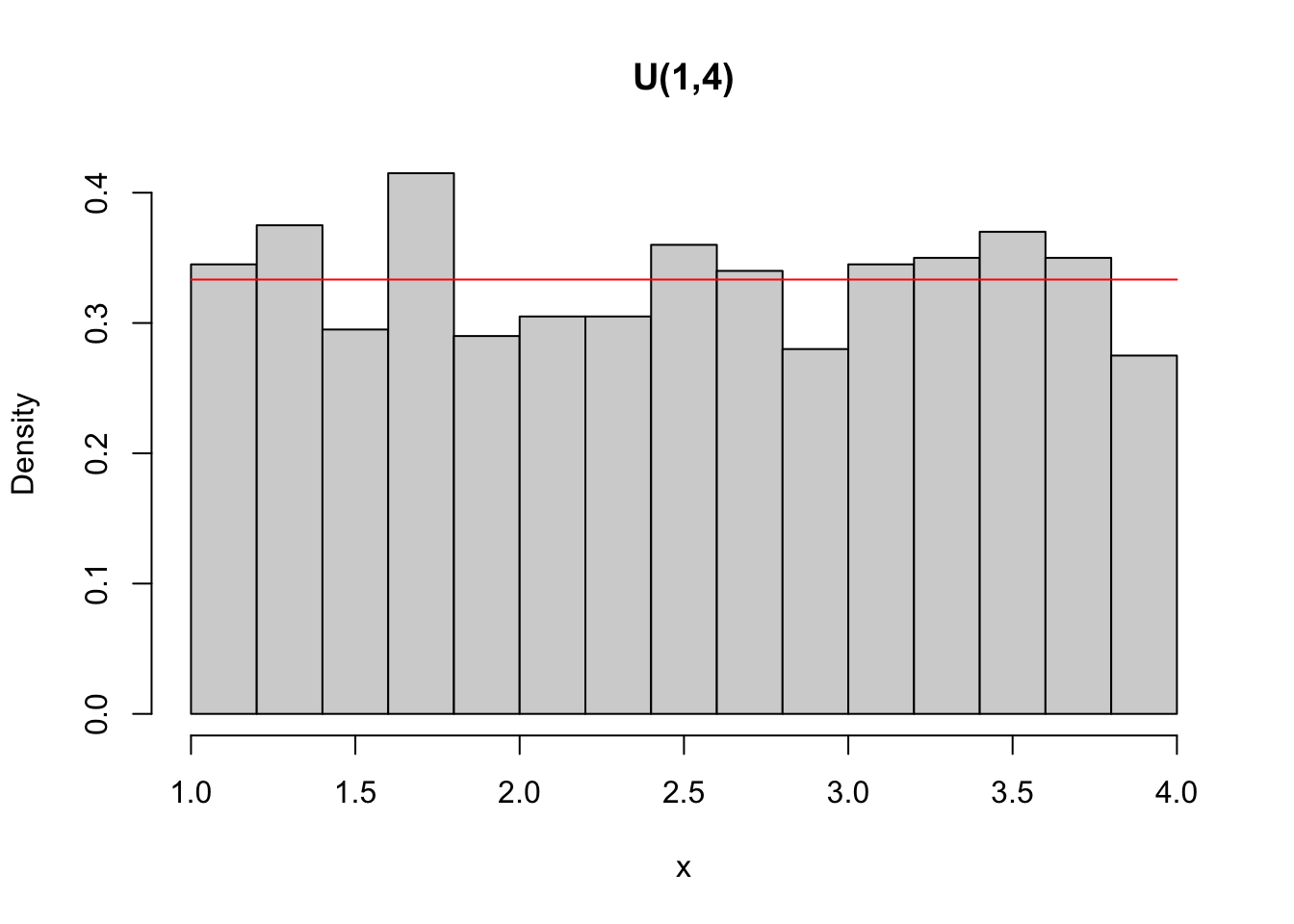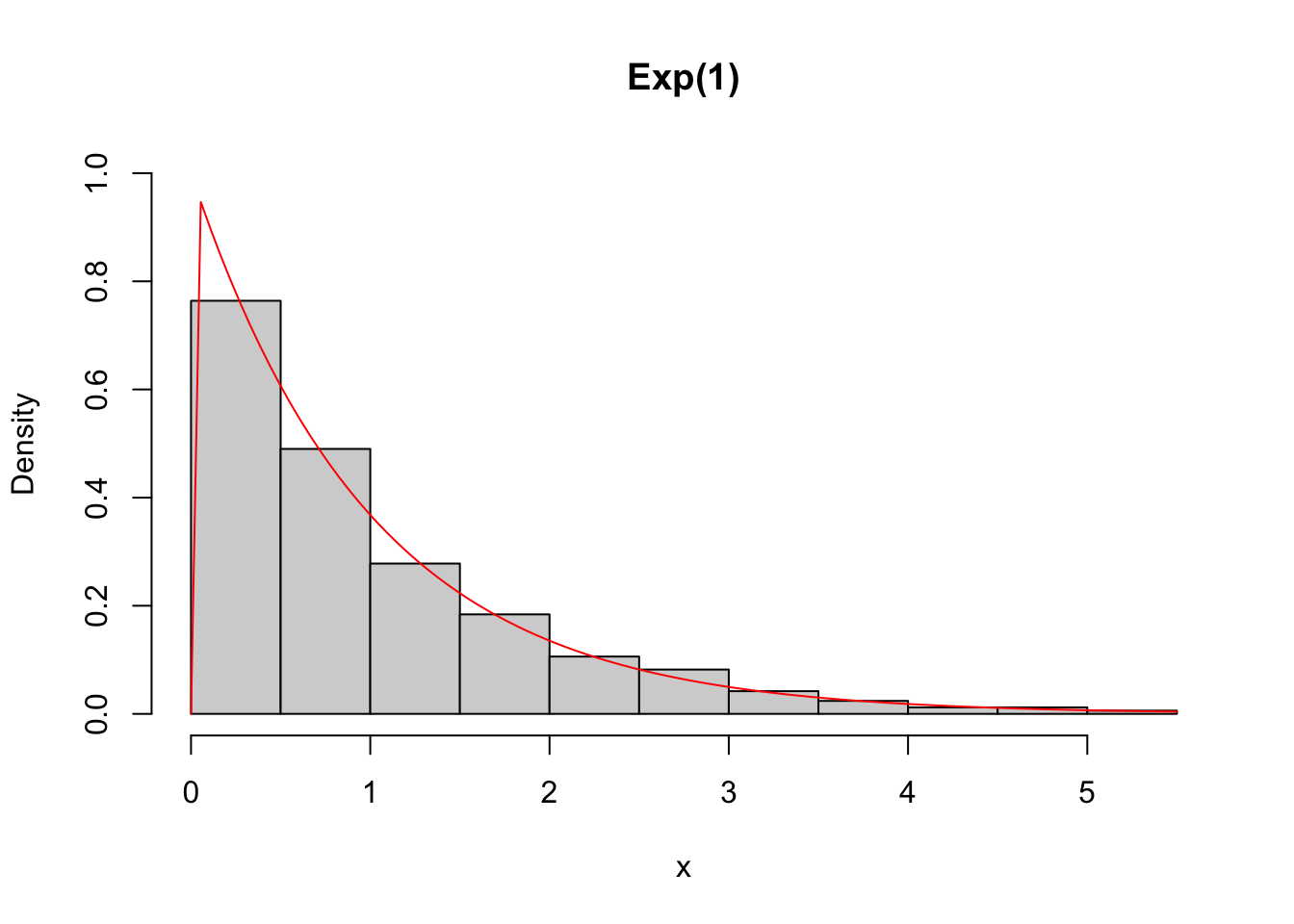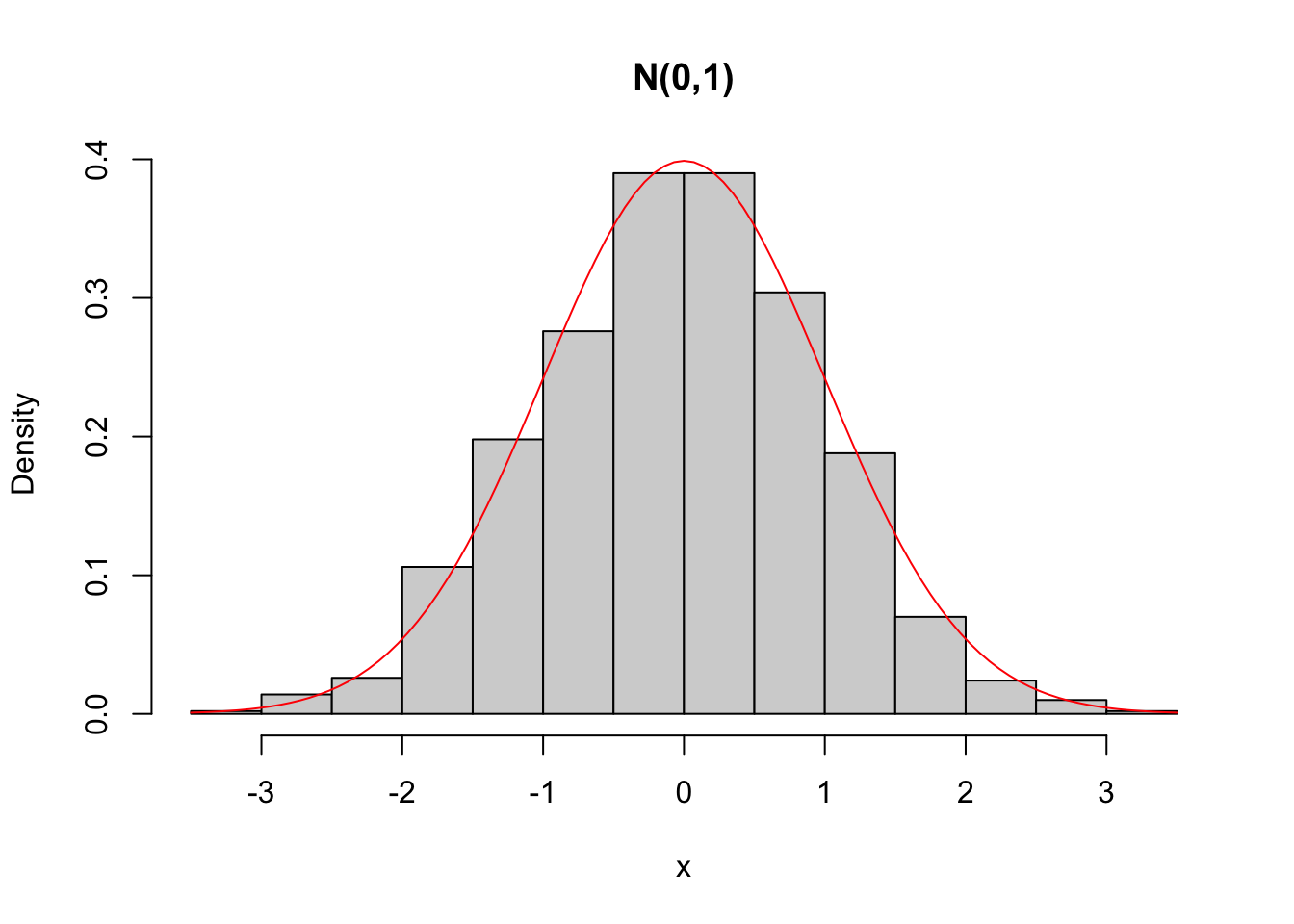## 3.9 Special Continuous Distributions

For more details, and are recommended. McLaughlin (2016) provides a compendium of probability distributions.

### 3.9.1Continuous Uniform$$\cdot \; \mathcal{U}(a,b)$$

The continuous uniform distribution in the interval $$\left[ a,b \right]$$ has its (probability) density (function) defined by

$\begin{equation} f(x|a,b) = \dfrac{1}{b-a} \tag{3.72} \end{equation}$

where $$a \le x \le b$$, $$-\infty < a,b < \infty$$ with $$b>a$$. Cumulative distribution function

$\begin{equation} F(x|a,b) = Pr(X<x) = \dfrac{x-a}{b-a} \tag{3.73} \end{equation}$

Expected value $\begin{equation} E(X) = \dfrac{a+b}{2} \tag{3.74} \end{equation}$

Variance $\begin{equation} V(X) = \dfrac{(b-a)^2}{12} \tag{3.75} \end{equation}$

Exercise 3.21 Demonstrate the Equations (3.73), (3.74) and (3.75) considering the identities (3.76) and (3.77).

$\begin{equation} a^2 - b^2 = (a-b)(a+b) \tag{3.76} \end{equation}$

$\begin{equation} a^3 - b^3 = (a-b)(a^2+ab+b^2) \tag{3.77} \end{equation}$

### 3.9.2Normal$$\cdot \; \mathcal{N}(\mu,\sigma)$$

The normal or Gaussian distribution (named after Johann Carl Friedrich Gauss) is denoted by $$\mathcal{N}(\mu,\sigma)$$. Its pdf is given by $\begin{equation} f(x|\mu,\sigma) = \dfrac{1}{\sqrt{2\pi} \sigma} \exp \bigg\{ -\frac{1}{2} \left( \frac{x-\mu}{\sigma} \right) ^2 \bigg\} \tag{3.78} \end{equation}$

for $$-\infty < x < \infty$$, $$-\infty < \mu < \infty$$, $$\sigma > 0$$. The parameters $$\mu$$ and $$\sigma$$ can be calculated respectively by the Equations (2.8) and (2.26). The notation $$\exp\{...\}$$ represents the Euler number raised to the expression enclosed by the square brackets.

The standard normal distribution is given by the expression $\begin{equation} f(z|\mu=0, \sigma=1) = \dfrac{1}{\sqrt{2\pi}} \exp \bigg\{ -\frac{z^2}{2} \bigg\} \tag{3.79} \end{equation}$

for $$-\infty < z < \infty$$.

Example 3.36 The normal distribution can be operated with the functions dnorm (density), pnorm (cumulative probability), qnorm (quantile) and rnorm (random/random) from the stats library.

# Pr(X < 2) in a N(0,1)
pnorm(2)
##  0.9772499
# quantile that limits 2.5% in a N(0,1)
qnorm(0.025)
##  -1.959964
# simulating 1000 pseudo-random values of a N(0,1)
set.seed(999); x <- rnorm(1000)
summary(x)
##      Min.   1st Qu.    Median      Mean   3rd Qu.      Max.
## -3.073370 -0.705544 -0.009566 -0.032516  0.636537  3.061137
# density of a N(0,1), overlapping simulated values
hist(x, freq = F,main = 'N(0,1)')
curve(dnorm(x), add = T, col = 'red')Exercise 3.22 Watch the video But what is the Central Limit Theorem? from the channel 3Blue1Brown. I thank Vitor Luiz Cavagnolli Machado for the suggestion.

Exercise 3.24 Consider the random variable $$Z$$ with standard normal distribution denoted by $$Z \sim \mathcal{N}(0,1)$$. Obtain the following quantities:
a. $$Pr(Z \le -1.96)$$.
b. $$Pr(-1 \le Z \le 1)$$.
c. $$Pr(Z > 1.64)$$.
d. $$q$$ tal que $$Pr(Z \le q) = 0.025$$.
e. $$q$$ tal que $$Pr(-q \le Z \le q) = 0.6826894921$$.
f. $$q$$ tal que $$Pr(Z > q) = 0.05$$.

Solution: Chapter ??
$$\\$$

Exercise 3.25 Refrigerators produced by a factory have a certain lifespan until the first damage. Studies indicate that this time follows a normal distribution with a mean of 1.45 years and a standard deviation of 0.15 years.

1. The factory offers a 1-year warranty. What is the probability of a refrigerator breaking down during this period?
2. How likely is it that a refrigerator will fail outside of warranty?
3. What is the probability of a refrigerator failing between the first and second year of use?
4. What is the probability of a refrigerator lasting more than 2 years without failing?
5. If the factory produced 80 thousand refrigerators, how many people should claim the warranty? $$\\$$

#### To know more

bring a collection of results and properties related to the normal distribution. provides a comprehensive treatment of results related to the multivariate normal distribution. The main themes are dependence, probability inequalities and their roles in theory and applications.

### 3.9.3Exponential$$\cdot \; \mathcal{E}(\lambda)$$

Consider again the toll described in Section 3.7.4, where on average $$\lambda$$ vehicles pass per minute. You can invert the reading, placing the time between each car as the new variable of interest. Thus, 1 car passes through this toll every $$\frac{1}{\lambda}$$ minutes. The continuous random variable $$X$$: ‘time between vehicles’ has exponential distribution of parameter $$\lambda$$, denoted by $X \sim \mathcal{E}(\lambda),$ where $$x > 0$$ and $$\lambda > 0$$ indicates the rate. The exponential density function is given by

$\begin{equation} f(x|\lambda) = \lambda e^{-\lambda x} \tag{3.80} \end{equation}$ where $$e$$ is the Euler’s number. Its cumulative distribution function is given by $\begin{equation} F(x|\lambda) = Pr(X \le x) = 1 - e^{-\lambda x} \tag{3.81} \end{equation}$ The expected value a nd variance are given by $\begin{equation} E(X)= \frac{1}{\lambda} = \lambda^{-1} \tag{3.82} \end{equation}$ $\begin{equation} V(X)=\frac{1}{\lambda^2} = \lambda^{-2} \tag{3.83} \end{equation}$

Example 3.37 The exponential distribution can be operated with the functions dexp (density), pexp (accumulated probability), qexp (quantile) and rexp (random/random) from the stats library.

# Pr(X < 2) in an exponential of rate 1
pexp(2, 1)
##  0.8646647
# quantile that limits 2.5% in an exponential of rate 1
qexp(0.025, 1)
##  0.02531781
# simulating 1000 pseudo random values of an exponential of rate 1
set.seed(999); x <- rexp(1000, 1)
summary(x)
##    Min. 1st Qu.  Median    Mean 3rd Qu.    Max.
## 0.00011 0.29347 0.69805 1.00508 1.41511 5.42528
# density of an exponential of rate 1, overlapping simulated values
hist(x, freq = F, ylim = c(0,1), main = 'Exp(1)')
curve(dexp(x,1), add = T, col = 'red')Example 3.38 Consider a tollbooth where an average of $$\lambda = 2$$ vehicles pass per minute. Thus, $X \sim \mathcal{E}(2),$ $f(x) = 2 e^{-2 x},$ $E(X)=\dfrac{1}{2}=0.5,$ $V(X)=\dfrac{1}{2^2}=0.25,$ $D(X) = \sqrt{0.25} = 0.5.$

Exercise 3.26 Considering the data from Example 3.38:
a. Define $$F(x)$$.
b. Get $$Pr(X<1)$$.
c. Obtain $$Pr(X>2)$$.
d. Sketch the graph of $$f(x)$$. $$\\$$

Exercise 3.27 Consider the exponential density given by Eq. (3.80), the definitions (3.69), (3.70) and @ref(eq: var-vad-x).
a. Show that $$F(x) = 1-e^{-\lambda x}$$.
b. Show that $$E(X) = \lambda^{-1}$$.
c. Show that $$V(X) = \lambda^{-2}$$. $$\\$$

### 3.9.4Student’s $$t$$$$\cdot \; \mathcal{t_\nu}$$

If $$X \sim t_\nu$$, then its pdf is given by

$\begin{equation} f(x|\nu) = \frac{\Gamma \left( \frac{\nu+1}{2} \right)}{\Gamma \left( \frac{\nu}{2} \right) \sqrt{\nu \pi}} \left( 1+\frac{x^2}{\nu} \right)^{-\frac{\nu + 1}{2}} \tag{3.84} \end{equation}$

where $$-\infty < x < \infty$$, $$\nu > 0$$ indicates the degrees of freedom and $$\Gamma$$ indicates the gamma function such that

$\begin{equation} \Gamma(x) = \int_{0}^{\infty} t^{x-1} e^{-t} dt \tag{3.85} \end{equation}$

Equivalently, one can write down $$X \sim t(\nu)$$.

Example 3.39 The $$t$$ distribution can be operated with the functions dt (density), pt (accumulated probability), qt (quantile) and rt (random/random) from the stats library.

# Pr(X < 2) in a t with 1 df
pt(2, 1)
##  0.8524164
# quantile that limits 2.5% in a t with 1 df
qt(0.025, 1)
##  -12.7062
# simulating 1000 pseudo random values from a t with 1 df
set.seed(246); x <- rt(1000, 1)
summary(x)
##      Min.   1st Qu.    Median      Mean   3rd Qu.      Max.
## -305.1952   -1.1336   -0.0891    0.3420    1.0430 1294.5135
# density of a t with 1 degree of freedom, overlapping simulated values
hist(x, 3000, freq = F, xlim = c(-15,15), main = expression(italic('t')(1)))
curve(dt(x,1), add = T, col = 'red')### 3.9.5Chi-squared$$\cdot \; \mathcal{\chi}^2_\nu$$

If $$X \sim \chi^2_\nu$$, then its pdf is given by

$\begin{equation} f(x|\nu) = \frac{1}{\Gamma \left( \frac{\nu}{2} \right) 2^{\nu/2}} x^{\frac{\nu}{2}-1} e^{-\frac{x}{2}} \tag{3.86} \end{equation}$

where $$x > 0$$, $$\nu > 0$$ indicates the degrees of freedom and $$\Gamma$$ is the gamma function according to Eq. (3.85). Equivalently, one can write down $$X \sim \chi^2(\nu)$$.

Example 3.40 The $$\chi^2$$ distribution can be operated with the functions dchisq (density), pchisq (accumulated probability), qchisq (quantile) and rchisq (random/random) from the stats library.

# Pr(X < 2) in a \chi^2 with 1 df
gl <- 1
pchisq(2, gl)
##  0.8427008
# quantile that  limits 2.5% in a \chi^2 with 1 df
qchisq(0.025, gl)
##  0.0009820691
# simulating 1000 pseudo random values from a \chi^2 with 1 df
set.seed(135); x <- rchisq(1000, gl)
summary(x)
##    Min. 1st Qu.  Median    Mean 3rd Qu.    Max.
## 0.00000 0.08634 0.42728 0.98606 1.30768 8.91451
# density of a \chi^2 with 1 degree of freedom, overlapping simulated values
hist(x, 50, freq = F, main = bquote(chi^2~  (.(gl))))
curve(dchisq(x,1), 0, 10, add = T, col = 'red')#### Characterization

$$Q \sim \chi^2_\nu$$ if

$\begin{equation} Q = \sum_{i=1}^\nu Z_i^2 \tag{3.87} \end{equation}$

where $$Z_i^2 \sim \mathcal{N}(0,1)$$, $$i \in \{1,\ldots,\nu\}$$.

Example 3.41 It is possible to simulate a chi-square with 1 degree of freedom.

# via standard normal squared
set.seed(1234); q1 <- rnorm(1000)^2
# via rchisq
set.seed(5678); q2 <- rchisq(1000, 1)
par(mfrow=c(1,2))
hist(q1, 30)
hist(q2, 30)# checking the adherence of the simulations
ks.test(q1, q2)
##
##  Asymptotic two-sample Kolmogorov-Smirnov test
##
## data:  q1 and q2
## D = 0.026, p-value = 0.8879
## alternative hypothesis: two-sided

Exercise 3.28 Simulate a chi-square with 3 degrees of freedom via Eq. (3.87). Compare with the simulation obtained via rchisq.

### 3.9.6Fisher-Snedecor$$\cdot \; \mathcal{F}_\nu$$

If $$X \sim \mathcal{F}_{\nu_1,\nu_2}$$, then its pdf is given by

$\begin{equation} f(x|\nu_1,\nu_2) = \frac{\sqrt{\frac{(\nu_1 x)^{\nu_1} \nu_2^{\nu_2}}{(\nu_1 x+\nu_2)^{\nu_1 + \nu_2}}}}{x B\left( \frac{\nu_1}{2}, \frac{\nu_2}{2} \right)} \tag{3.88} \end{equation}$

where $$x > 0$$, $$\nu_1 > 0$$ indicates the numerator degrees of freedom, $$\nu_2 > 0$$ indicates the denominator degrees of freedom and $$B$$ indicates the beta function, such that

$\begin{equation} B(x_1,x_2) = \int_{0}^{1} t^{x_1 - 1} (1-t)^{x_2 - 1} dt = \frac{\Gamma(x_1) \Gamma(x_2)}{\Gamma(x_1+x_2)} \tag{3.89} \end{equation}$

Example 3.42 The distribution $$\mathcal{F}$$ can be operated with the functions df (density), pf (cumulative probability), qf (quantile) and rf (random/random) from the library stats.

# Pr(X < 2) in an F with 1 df in the numerator and 3 df in the denominator
gl1 <- 1
gl2 <- 3
pf(2, gl1, gl2)
##  0.7477845
# quantile that limits 2.5% in an F(1,3)
qf(0.025, gl1, gl2)
##  0.001157189
#simulating 1000 pseudo-random values of an F(1,3)
set.seed(1010); x <- rf(1000, gl1, gl2)
summary(x)
##     Min.  1st Qu.   Median     Mean  3rd Qu.     Max.
##   0.0000   0.1073   0.5564   2.3601   1.9758 120.0880
# density of an F(1,3), overlapping simulated values
hist(x, 500, freq = F, xlim = c(0,10), main = bquote(F(.(gl1),.(gl2))))
curve(df(x, gl1, gl2), 0, 10, add = TRUE, col = 'red')#### Characterization

$$X \sim \mathcal{F}_{\nu_1,\nu_2}$$ if

$\begin{equation} X = \frac{Q_1/\nu_1}{Q_2/\nu_2} \tag{3.90} \end{equation}$

where $$Q_1 \sim \chi^2_{\nu_1}$$ and $$Q_2 \sim \chi^2_{\nu_2}$$.

Example 3.43 It is possible to simulate a $$\mathcal{F}$$ with $$\nu_1=1$$ degree of freedom in the numerator and $$\nu_2=3$$ degrees of freedom in the denominator.

set.seed(1234); q1 <- rchisq(1000, 1)
set.seed(5678); q2 <- rchisq(1000, 3)
x1 <- (q1/1)/(q2/3)
x2 <- rf(1000, 1, 3)
par(mfrow=c(1,2))
hist(x1, 50, xlim = c(0,20))
hist(x2, 100, xlim = c(0,20))ks.test(x1, x2)
##
##  Asymptotic two-sample Kolmogorov-Smirnov test
##
## data:  x1 and x2
## D = 0.023, p-value = 0.9541
## alternative hypothesis: two-sided

### 3.9.7Beta$$\cdot \; \mathcal{Beta}(\alpha,\beta)$$

The beta density function is given by $\begin{equation} f(x|\alpha,\beta) = \dfrac{\Gamma(\alpha+\beta)}{\Gamma(\alpha)\Gamma(\beta)} x^{\alpha-1} (1-x)^{\beta-1} \tag{3.91} \end{equation}$ where $$0 \le x \le 1$$, $$\alpha,\beta > 0$$ and $$\Gamma$$ is the gamma function according to Eq. (3.85). Expected value and variance are given by $\begin{equation} E(X) = \frac{\alpha}{\alpha+\beta} \tag{3.92} \end{equation}$ $\begin{equation} V(X) = \frac{\alpha\beta}{(\alpha+\beta)^2 (\alpha+\beta+1)} \tag{3.93} \end{equation}$

The median and mode are given by $\begin{equation} Md(X) \approx \frac{\alpha-1/3}{\alpha+\beta-2/3} \tag{3.94} \end{equation}$ $\begin{equation} Mo(X) = \frac{\alpha-1}{\alpha+\beta-2}, \; \alpha,\beta>1 \tag{3.95} \end{equation}$ $\begin{equation} Mo(X) \; \text{algum valor entre 0 e 1}, \; \alpha=\beta=1 \tag{3.96} \end{equation}$ $\begin{equation} Mo(X) = \{0,1\}, \; \alpha,\beta<1 \tag{3.97} \end{equation}$ $\begin{equation} Mo(X) = 0, \; \alpha \le 1,\beta>1 \tag{3.98} \end{equation}$ $\begin{equation} Mo(X) = 1, \; \alpha>1,\beta \le 1 \tag{3.99} \end{equation}$

Example 3.44 The Beta distribution can be operated with the functions dbeta (density), pbeta (cumulative probability), qbeta (quantile) and rbeta (random/random) from the stats library.

# Pr(X < 1/2) in a Beta(1,3)
pbeta(1/2, 1, 3)
##  0.875
# quantile that limits 2.5% in a Beta(1,3)
qbeta(0.025, 1, 3)
##  0.008403759
# simulating 1000 pseudo random values of a Beta(1,3)
set.seed(1010); x <- rbeta(1000, 1, 3)
summary(x)
##      Min.   1st Qu.    Median      Mean   3rd Qu.      Max.
## 0.0000842 0.0905449 0.2126896 0.2502407 0.3621014 0.9394182
# density of a Beta(1,3), overlaying simulated values
hist(x, 30, freq = F, ylim = c(0, 3), main = 'Beta(1,3)')
curve(dbeta(x,1,3), add = T, col = 'red')### 3.9.8Gamma$$\cdot \; \mathcal{Gama}(k,g)$$

The gamma density function can be given by $\begin{equation} f(x|k,g) = \frac{1}{\Gamma(k) g^k} x^{k-1} e^{-\frac{x}{g}} \tag{3.100} \end{equation}$ where $$x>0$$, $$k>0$$ (shape), $$g>0$$ (scale) and $$\Gamma$$ is the gamma function according to Eq. (3.85). Expected value and variance are given by $\begin{equation} E(X) = kg \tag{3.101} \end{equation}$ $\begin{equation} V(X) = kg^2 \tag{3.102} \end{equation}$

The median does not have a closed form, and the mode is given by $\begin{equation} Mo(X) = (k-1)g, \;\; k \ge 1 \tag{3.103} \end{equation}$ $\begin{equation} Mo(X) = 0, \;\; k < 1 \tag{3.103} \end{equation}$

Example 3.45 The Gamma distribution can be operated with the functions dgamma (density), pgamma (accumulated probability), qgamma (quantile) and rgamma (random/random) from the stats library.

# Pr(X < 2) in a Gamma(1,3)
pgamma(2, 1, 3)
##  0.9975212
# quantile that limits 2.5% in a Gamma(1,3)
qgamma(0.025, 1, 3)
##  0.008439269
# simulating 1000 pseudo-random values of a Gamma(1,3)
set.seed(1010); x <- rgamma(1000, 1, 3)
summary(x)
##      Min.   1st Qu.    Median      Mean   3rd Qu.      Max.
## 0.0003625 0.0914895 0.2279837 0.3381387 0.4639010 2.6271045
# density of a Gamma(1,3), overlapping simulated values
hist(x, 30, freq = F, ylim = c(0, 3), main = 'Gama(1,3)')
curve(dgamma(x,1,3), add = T, col = 'red')Exercise 3.29 Consider the following parameterization of the Gamma distribution: $\begin{equation} f(x) = \frac{\beta^\alpha}{\Gamma(\alpha)} x^{\alpha-1} e^{-\beta x} \tag{3.104} \end{equation}$ where $$x>0$$, $$\alpha>0$$ (shape) and $$\beta>0$$ (rate).

1. Check that the shape parameter $$k$$ of Eq. (3.100) is equal to the shape parameter $$\alpha$$ of Eq. (3.104).
2. Check that the scale parameter $$g$$ of Eq. (3.100) is the inverse of the rate $$\beta$$ of Eq. (3.104).

### 3.9.9Triangular$$\cdot \; \mathcal{Tri}(a,m,b)$$

define the triangular density function in the interval $$[a,b]$$ with mode $$m$$ by $\begin{equation} f(x|a,b,m) = \left\{ \begin{array}{l} \frac{2}{b-a} \frac{x-a}{m-a}, \;\; a \le x \le m \\ \frac{2}{b-a} \frac{b-x}{b-m}, \;\; m < x \le b \\ \end{array} \right. \tag{3.105} \end{equation}$

where $$a \le x \le b$$ e $$a \le m \le b$$, $$-\infty < a,b < \infty$$ with $$b>a$$.

Its cumulative distribution function is given by $\begin{equation} F(x|a,b,m) = Pr(X \le x) = \left\{ \begin{array}{l} \frac{m-a}{b-a} \left( \frac{x-a}{m-a} \right)^2, \;\; a \le x \le m \\ 1 - \frac{b-m}{b-a} \left( \frac{b-x}{b-m} \right)^2, \;\; m < x \le b \\ \end{array} \right. \tag{3.106} \end{equation}$

Its inverse cumulative distribution function is given by $\begin{equation} F^{-1}(u|a,b,m) = \left\{ \begin{array}{l} a + \sqrt{u(m-a)(b-a)}, \;\; 0 \le u \le \frac{m-a}{b-a} \\ b - \sqrt{(1-u)(b-m)(b-a)}, \;\; \frac{m-a}{b-a} < u \le 1 \\ \end{array} \right. \tag{3.107} \end{equation}$

presents the EnvStats package, which has varied functions for Environmental Statistics that consider the triangular distribution.

library(EnvStats)
set.seed(2); hist(rtri(10000), 40, freq = FALSE, main = 'Tri(0,1/2,1)')
curve(dtri(x), col = 'red', add = TRUE)Exercise 3.30 See the documentation for ?EnvStats::dtri.

### 3.9.10Gompertz$$\cdot \; \mathcal{Gompertz}(\alpha,\beta)$$

defines the Gompertz density function of shape parameter $$\alpha>0$$ and scale $$\beta>0$$ for $$x>0$$ by $\begin{equation} f(x|\alpha,\beta) = \alpha \beta \exp \left\{ \beta x + \alpha - \alpha e^{\beta x} \right\} \tag{3.108} \end{equation}$

Its cumulative distribution function is given by $\begin{equation} F(x|\alpha,\beta) = 1 - \exp \left\{ - \alpha(e^{\beta x}-1) \right\} \tag{3.109} \end{equation}$

(Yee 2010) presents functions for the Gompertz distribution.

library(VGAM)
curve(dgompertz(x, scale = 1, shape = .1), xlim = c(0,5), ylim = c(0,1.2),
col = 'red')
curve(dgompertz(x, scale = 1, shape = 2), xlim = c(0,5), ylim = c(0,1.2),
col = 'black', add = TRUE)
curve(dgompertz(x, scale = 1, shape = 3), xlim = c(0,5), ylim = c(0,1.2),
col = 'blue', add = TRUE)
curve(dgompertz(x, scale = 2, shape = 1), xlim = c(0,5), ylim = c(0,1.2),
col = 'green', add = TRUE)### 3.9.11 Unit-Gompertz $$\cdot \; \mathcal{GU}(\alpha,\beta)$$

define the Unit-Gompertz distribution from the type transformation $X=e^{-Y}$

where $$Y$$ has a Gompertz distribution. Its density function with parameters $$\alpha>0$$ and $$\beta>0$$ with $$0<x<1$$ is given by

$\begin{equation} f(x|\alpha,\beta) = \alpha \beta x^{-(\beta+1)} \exp \left\{ -\alpha(x^{-\beta}-1) \right\} \tag{3.110} \end{equation}$

Its cumulative distribution function is given by $\begin{equation} F(x|\alpha,\beta) = \exp \left\{ -\alpha(x^{-\beta}-1) \right\} \tag{3.111} \end{equation}$

present the unitquantreg package, that provides a collection of parametric quantile regression models for bounded data. The autors also present functions for the unit-Gompertz distribution reparametrized in terms of the $$\tau$$-th quantile, $$\tau \in (0,1)$$.

library(unitquantreg)
set.seed(123)
x <- rugompertz(n = 5000, mu = 0.5, theta = 2, tau = 0.5)
R <- range(x)
S <- seq(from = R, to = R, by =  0.01)
hist(x, prob = TRUE, main = 'Gompertz unit')
lines(S, dugompertz(x = S, mu = 0.5, theta = 2, tau = 0.5), col = 2)plot(ecdf(x))
lines(S, pugompertz(q = S, mu = 0.5, theta = 2, tau = 0.5), col = 2)plot(quantile(x, probs = S), type = "l")
lines(qugompertz(p = S, mu = 0.5, theta = 2, tau = 0.5), col = 2)### 3.9.12 Continuous Poisson

presents and discusses continuous counterparts of the Poisson and binomial distributions.

### References

Gompertz, Benjamin. 1825. “On the Nature of the Function Expressive of the Law of Human Mortality, and on a New Mode of Determining the Value of Life Contingencies. In a Letter to Francis Baily, Esq. FRS &c.” Philosophical Transactions of the Royal Society of London, no. 115: 513–83. https://royalsocietypublishing.org/doi/pdf/10.1098/rstl.1825.0026.
Ilienko, Andrii. 2013. “Continuous Counterparts of Poisson and Binomial Distributions and Their Properties.” arXiv Preprint arXiv:1303.5990. https://arxiv.org/abs/1303.5990.
Johnson, Norman L, Samuel Kotz, and Narayanaswamy Balakrishnan. 1994. Continuous Univariate Distributions. 2nd ed. John Wiley & Sons.
———. 1995. Continuous Univariate Distributions. 2nd ed. Vol. 2. John Wiley & Sons.
Kotz, Samuel, and Johan René Van Dorp. 2004. Beyond Beta: other continuous families of distributions with bounded support and applications. World Scientific. https://doi.org/10.1142/5720.
Mazucheli, Josmar, André Felipe Menezes, and Sanku Dey. 2019. “Unit-Gompertz Distribution with Applications.” Statistica 79 (1): 25–43. https://rivista-statistica.unibo.it/article/download/8497/9401.
Menezes, Andr’e F. B., and Josmar Mazucheli. 2021. Unitquantreg: Parametric Quantile Regression Models for Bounded Data. https://andrmenezes.github.io/unitquantreg/.
Millard, Steven P. 2013. EnvStats: An R Package for Environmental Statistics. New York: Springer. https://link.springer.com/book/9781461484554.
Patel, Jagdish K., and Campbell B. Read. 1982. “Handbook of the Normal Distribution.” Marcel Dekker, New York.
Tong, Yung L. 1990. The Multivariate Normal Distribution. Springer-Verlag New York Inc.
Yee, Thomas W. 2010. “The VGAM Package for Categorical Data Analysis.” Journal of Statistical Software 32 (10): 1–34. https://doi.org/10.18637/jss.v032.i10.